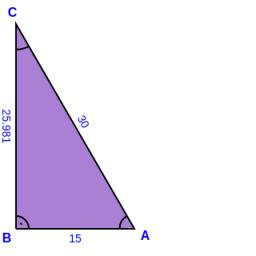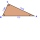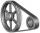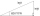# Perimeter of triangle

In the triangle, ABC angle A is 60° angle B is 90°, and side size c is 15 cm. Calculate the triangle circumference.

o =  70.9808 cm

### Step-by-step explanation:

Try calculation via our triangle calculator.Did you find an error or inaccuracy? Feel free to write us. Thank you!Tips to related online calculators
Pythagorean theorem is the base for the right triangle calculator.

#### You need to know the following knowledge to solve this word math problem:

We encourage you to watch this tutorial video on this math problem:

## Related math problems and questions:

• Right triangle trigonometricsCalculate the size of the remaining sides and angles of a right triangle ABC if it is given: b = 10 cm; c = 20 cm; angle alpha = 60° and the angle beta = 30° (use the Pythagorean theorem and functions sine, cosine, tangent, cotangent)
• 30-60-90The longer leg of a 30°-60°-90° triangle measures 5. What is the length of the shorter leg?
• CalculateCalculate the area of triangle ABC, if given by alpha = 49°, beta = 31°, and the height on the c side is 9cm.
• MedianIn triangle ABC is given side a=10 cm and median ta= 13 cm and angle gamma 90°. Calculate length of the median tb.
• The right triangleIn the right triangle ABC with a right angle at C, we know the side lengths AC = 9 cm and BC = 7 cm. Calculate the length of the remaining side of the triangle and the size of all angles.
• CloudsApproximately at what height is the cloud we see under an angle of 26°10' and see the Sun at an angle of 29°15' and the shade of the cloud is 92 meters away from us?
• Right angleIn a right triangle ABC with a right angle at the apex C, we know the side length AB = 24 cm and the angle at the vertex B = 71°. Calculate the length of the legs of the triangle.
• RectangularRectangular triangle KLM with right angle at vertex L, angle beta at vertex K and angle alpha at vertex M. Angle at vertex M = 65°, side l = 17.5 cm. Use Pythagorean theorems and trigonometric functions to calculate the lengths of all sides and the angle
• Inner anglesThe inner angles of the triangle are 30°, 45° and 105° and its longest side is 10 cm. Calculate the length of the shortest side, write the result in cm up to two decimal places.
• NonagonCalculate the area and perimeter of a regular nonagon if its radius of the inscribed circle is r = 10cm
• Pyramid 8Calculate the volume and the surface area of a regular quadrangular pyramid with the base side 9 cm and side wall with the base has an angle 75°.
• Triangle SAAThe triangle has one side long 71 m and its two internal angles is 60°. Calculate the perimeter and area of the triangle.
• V-beltCalculate the length of the belt on pulleys with diameters of 105 mm and 393 mm at shaft distance 697 mm.
• Right triangleIt is given a right triangle angle alpha of 90 degrees beta angle of 55 degrees c = 10 cm use Pythagorean theorem to calculate sides a and b
• Triangle - is RT?Triangle has a circumference of 90 cm. Side b is 1 cm longer than c, and side c is 31 cm longer than side a. Calculate the length of sides and determine whether a triangle is a right triangle.
• Area and two anglesCalculate the size of all sides and internal angles of a triangle ABC, if it is given by area S = 501.9; and two internal angles α = 15°28' and β = 45°.
• One sideOne side is 36 long with a 15° incline. What is the height at the end of that side?